Excel Solver with a Simple Example (Step by Step)

In this article, we’re going to use a simple example to introduce you to Solver technique and then in next article, we’ll present some complex examples to explain what this feature can do for you.

The following figure shows a worksheet. It is a set up to calculate the profit for three products.

Our Hypothetical Problem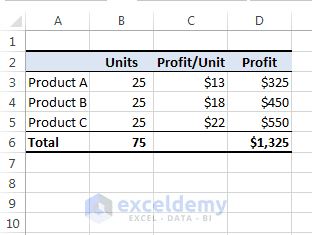We’ll use Solver to find out the number of units to maximize the total profit.

Column B displays the number of units of each product. Profit per unit for each product is shown in Column C, and Column D contains formulas. These formulas calculate the total profit for each product by multiplying the units by the profit per unit.

You don’t need to be a business graduate to realize that the highest profit comes from Product C. Therefore, to maximize total profit from production, the production management can produce only Product C. But if things were so straightforward, you wouldn’t need tools like Solver. This company has some constraints that must be met to produce products:

• The combined production capacity is 300 units per day.
• The company needs 50 units of Product A to fill an existing order.
• The company needs 40 units of Product B to fill an expected order.
• The market for Product C is relatively limited. So the company is not interested in producing more than 40 units of this product per day.

Read More: Resource Allocation Model in Excel Using Solver

These four constraints make it a real problem and a bit more challenging. In fact, it’s a perfect problem for using Solver technique.

The procedure of using Excel Solver

Before going more detail, here’s the basic procedure for using Solver:

• First of all, set up the worksheet with values and formulas. Make sure that you have formatted cells correctly; for example, maximum time you can’t produce partial units of your products, so format those cells to contain numbers with no decimal values.
• Choose Data ➪ Analysis ➪ Solver. The Solver Parameters dialog box will appear.
• Specify the target cell. Target cell also is known as objective.
• Specify the range that contains the changing cells.
• Specify the constraints.
• If necessary, change the Solver options.
• Let Solver solve the problem.

Tackling this example

To solve this example using Solver, choose Data ➪ Analysis ➪ Solver. The Solver Parameters dialog box will appear. Set up this dialog box in the following steps to solve our problem.

In this example, the target cell is D6. D6 cell calculates the total profit for three products.

• Enter D6 in the Set Objective field of the Solver Parameters dialog box.
• We’re going to maximize the value of cell D6, so select the Max option button.
• Specify the changing cells in the By Changing Variable Cells field. Our changing cells are B3: B5.
• In this step, we’ll specify the constraints on the problem. The constraints are added one by one and appear in the Subject to the Constraints list. To add a constraint, click the Add button. The Add Constraint dialog box appears as shown in the following figure. This dialog box has three parts: a Cell Reference, an operator, and a Constraint value.
• To set the first constraint (our first constraint is: the total production capacity is 300 units), enter B6 as the Cell Reference, choose equal (=) from the drop-down list of operators, and enter 300 as the Constraint value.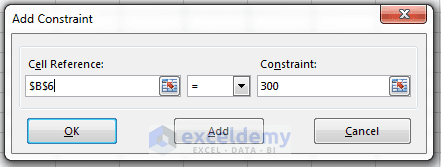• Click Add, and enter the remaining constraints. The following table summarizes the constraints for this problem.

Read More: Minimizing shipping costs in Excel using Solver

TABLE: Constraints Summary

ConstraintExpressed As
Capacity is 300 unitsB6=300
At least 50 units of Product AB3>=50
At least 40 units of Product BB4>=40
No more than 40 units of Product CB5<=40
• When we’ve finished entering our last constraint, we’ve clicked OK and we’re returned to the Solver Parameters dialog box. This dialog box now lists the four constraints.
• Now select Simplex LP solving method from the Select a Solving Method drop-down list.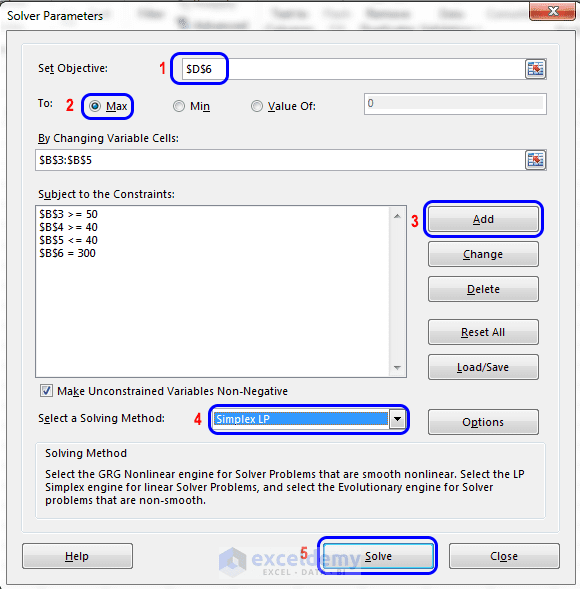The Solver Parameters dialog box. Steps are shown in this image.

• Click the Solve button to start the solution process. Excel will watch you the progress onscreen and soon announces that it has found a solution. The Solver Results dialog box is shown in the following figure.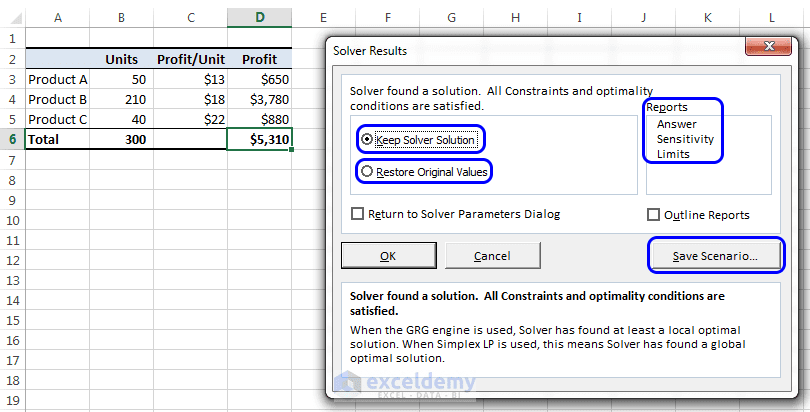Solver displays this Solver Result dialog box when it finds a solution to your problem.

At this point, we have the following options in our hand:

• You can keep the solution that Solver has found.
• You can restore the original changing cell values.
• You can create any or all of the three reports that show what Solver did.
• Click the Save Scenario button to save the solution as a scenario so that Scenario Manager can use it.

From the Reports section of the Solver Results dialog box, you can select any or all of three optional reports to produce reports. After selecting a report (or all), click OK. Excel will create each report on a new worksheet, with an appropriate name. The following figure shows an Answer Report.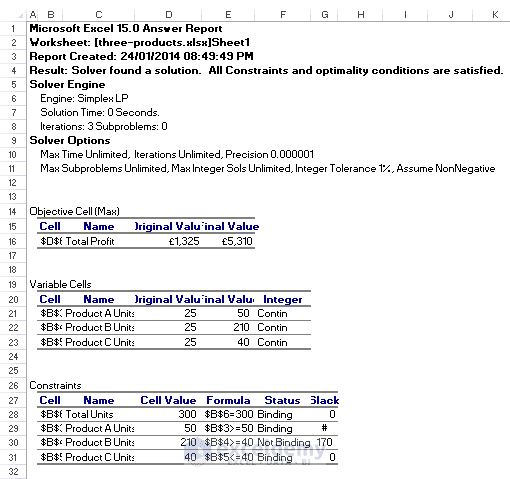Answer Report, one of three reports that Solver can produce.

Observe the Constraints section of the report. You will find that three of the four constraints are binding, which means that these constraints were satisfied at their limit.

With this simple example, we have understood how Solver works. Sometimes, you can solve this type of problem manually, but this is not always the case. For a complex problem, you’ve to depend on Solve technique of Excel.

Caution: You can’t undo any changes that Solver has made to your workbook.

Read More: What is Solver in Excel

We’re going to discuss the Solver Options dialog box in this section. Using this dialog box, you can control many aspects of the solution process. You can also load and save model specifications in a worksheet range using this dialog box.

Usually, you’ll want to save a model only when you will use more than one set of Solver parameters with your worksheet. Excel saves the first Solver model automatically with your worksheet using hidden names. If you save additional models, Excel stores the information in the form of formulas that correspond to the specifications. (The last cell in the saved range is an array formula that holds the options settings.)

It may happen that Solver will report it can’t find a solution, even when you know that one solution should exist. You can change one or more of the Solver options and try again. When you click the Options button in the Solver Parameters dialog box, the Solver Options dialog box shown in the following figure appears.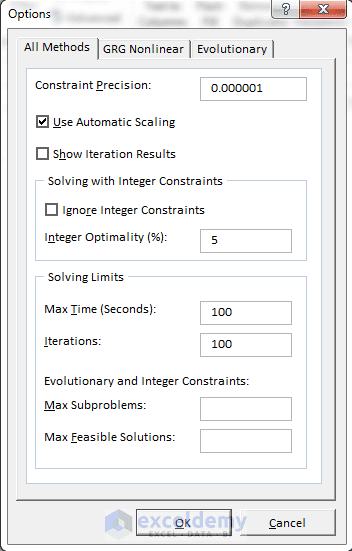We can control many aspects of how Solver will solve a problem.

Here is a brief description of Solver’s options:

• Constraint Precision: Specify how close the Cell Reference and Constraint formulas must be to satisfy a constraint. Specifying less precision will make Excel solve the problem more quickly.
• Use Automatic Scaling: It is used when the problem deals with large differences in magnitude— when you attempt to maximize a percentage, for example, by varying cells that are very large.
• Show Iteration Results: By selecting this checkbox, Solver is instructed to pause and display the results after each iteration.
• Ignore Integer Constraints: If you select this check box, Solver will ignore constraints that mention that a particular cell must be an integer. Using this option may permit Solver to find a solution that can’t be found otherwise.
• Max Time: Mention the maximum amount of time (in seconds) that you want Solver to spend on a single problem. If Solver reports that it exceeded the time limit, you can increase the amount of time that it will spend to search for a solution.
• Iterations: Enter the maximum number of trial solutions that you want Solver to try to solve the problem.
• Max Subproblems: It is used to solve complex problems. Specify the maximum number of sub-problems that may be solved by the Evolutionary algorithm.
• Max Feasible Solutions: It is used for complex problems. Specify the maximum number of feasible solutions that may be solved by the Evolutionary algorithm.
Note: The other two tabs in the Options dialog box contain additional options used by the GRG Nonlinear and Evolutionary Algorithms.

Happy Excelling 🙂

Kawser

Hello! Welcome to my Excel blog! It took me some time to be a fan of Excel. But now I am a die-hard fan of MS Excel. I learn new ways of doing things with Excel and share here. Not only how to guide on Excel, but you will get also topics on Finance, Statistics, Data Analysis, and BI. Stay tuned!